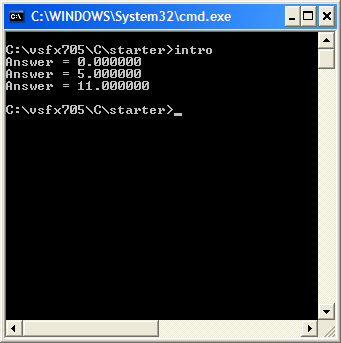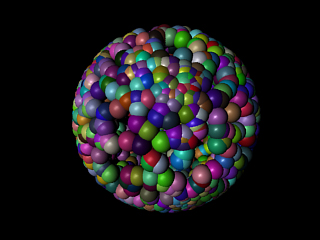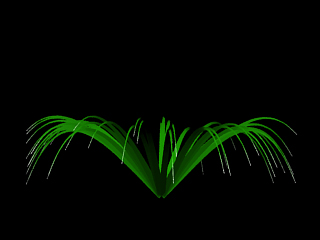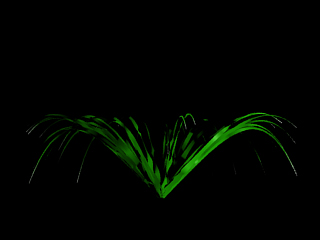C Programming

 #include float anotherAdd() { return 2 + 9; } void add(float *ptr) { *ptr = 2 + 3; } void main() { float answer = 0.0; printf("Answer = %f\n", answer); add(&answer); printf("Answer = %f\n", answer); answer = anotherAdd(); printf("Answer = %f\n", answer); }     This is a basic script is written to show the use of C procedures(main()) and pointers(*ptr). By executing this script we get a simple return of what is asked to be calculated. The script is run from the command line as to use the .exe that is created when the script is built. The pointer allows us to call to memory addresses that already store information that we might need. Script Returns#include <stdio.h>
#include <stdlib.h>
#include <math.h>
#include "C:/vsfx705/C/lib/library.h"

void main ()
{
char buffer;
float detail, radius, bbSize;
int n, num;

while (gets(buffer))

{
//Get the data from PRman

sscanf(buffer,"%f %d %f %f", &detail, &num, &radius, &bbSize);

//Get a random location within the bounding box
for(n = 0; n < num; n++)
{
float x = randBetween(-bbSize/2, bbSize/2);
float y = randBetween(-bbSize/2, bbSize/2);
float z = randBetween(-bbSize/2, bbSize/2);

//create a randomly translated sphere
printf("AttributeBegin\n");
printf("Color %f %f %f\n", randBetween(0,1), randBetween(0,1), randBetween(0,1));
printf("Translate %f %f %f\n", x, y, z);
printf("Sphere %f -%f %f 360\n", radius, radius, radius);
printf("AttributeEnd\n");
}

//Tell PRman that the procedure is done

printf("%c", '\377');
fflush(stdout);
}

}

This is the start of creating a procedure that will create random spheres within a specified boundary(bounding box). Shown here is an example of how this script works. By using "rand()" within the procedure randBetween in the library.c file, we are able to create any number of spheres with a set size within the boundary and add to them random RGB values. This is all utilized in Maya using MtoR and creating a SLIM Ribbox to run the procedure.

 library.c File   #include "C:/vsfx705/C/lib/library.h" #include #include #include //_______________________________________ float randBetween(float min, float max) { return (float)rand()/RAND_MAX * (max - min) + min;

Script Returnslibrary.h File   float randBetween(float min, float max);

#include <stdio.h>
#include <stdlib.h>
#include <math.h>

#include "H:/vsfx705/C/lib/library.h"

/*_________________________________*/
void sphereCloud(float radius, float bSize);
void normalize(float xyz[]);
float randBetween(float min, float max);
/*_________________________________*/
void normalize(float xyz[])
{
float xNorm, yNorm, zNorm, norm;

xNorm = xyz * xyz;
yNorm = xyz * xyz;
zNorm = xyz * xyz;

norm = xNorm + yNorm + zNorm;

norm = (float)sqrt(norm);

xyz /= norm;
xyz /= norm;
xyz /= norm;
}
/*_________________________________*/

void sphereCloud(float radius, float bSize)
{
float xyz;
printf("TransformBegin\n");
printf("Color %f %f %f\n", randBetween(0,1), randBetween(0,1), randBetween(0,1));
xyz = randBetween(-bSize/2, bSize/2);
xyz = randBetween(-bSize/2, bSize/2);
xyz = randBetween(-bSize/2, bSize/2);
normalize(xyz);
printf("Translate %f %f %f\n", xyz, xyz, xyz );
printf("Sphere %f %f %f 360\n", radius, -radius, radius);
printf("TransformEnd\n");
}
/*__________________________________________*/
void main()
{
float detail, bbox;
char buffer;
int n, num;
while(gets(buffer) /*!= NULL*/)
{
sscanf(buffer, "%f %f %d", &detail, &bbox, &num);
for(n=0; n < num; n++)
sphereCloud(bbox/10, bbox);
printf("%c", '\377');
fflush(stdout);
}
}

This more detailed script allow us to generate the same type of spheres within a boundary. But this takes it further by adjusting the coordinates of the spheres on the surface of the boundary instead of within the boundary. the "normalize" procedure allow this to happen.

 library.c File   #include "C:/vsfx705/C/lib/library.h" #include #include #include //_______________________________________ float randBetween(float min, float max) { return (float)rand()/RAND_MAX * (max - min) + min;

Script Returnslibrary.h File   float randBetween(float min, float max);

library.h File

// These reproduce the data types found in MEL
typedef struct {
float x, y, z;
} vector;

float randBetween(float min, float max);
vector subtract(vector a, vector b);
vector add(vector a, vector b);
vector scalar(vector a, float s);
float flerp(float a, float b, float pct);

Because of some of the limitations of C, we need to create our own library.c file to allow us to add, subtract, and scalar vectors. This file also includes are basic rand() function and a lerp function. Within the library.h file we are actually defining for C what a vector is, so that we can use similar ideas for the "plants" that we used in MEL.

 library.c File   #include "C:/vsfx705/C/lib/library.h" #include #include #include //_______________________________________ float randBetween(float min, float max) { return (float)rand()/RAND_MAX * (max - min) + min; } //_______________________________________ vector subtract(vector a, vector b) { static vector out; out.x = a.x - b.x; out.y = a.y - b.y; out.z = a.z - b.z; return out; } //_______________________________________ vector add(vector a, vector b) { static vector out; out.x = a.x + b.x; out.y = a.y + b.y; out.z = a.z + b.z; return out; } //_______________________________________ vector scalar(vector a, float s) { a.x *= s; a.y *= s; a.z *= s; return a; } //_______________________________________ float flerp(float a, float b, float pct) { return (1.0 - pct) * a + pct * b; }

 plant.h File   // Declarations of functions implemented in // plant.c #include "C:/vsfx705/C/lib/library.h" typedef struct { float pixels; int leafCount; int leafCv; float leafBase, leafTip; vector leafRGB; float leafX, leafY; float leafDroop; }PlantDB; void leaf(PlantDB plant, vector end); vector* subdivide(int num, vector B, vector E, float falloff);

The creation of a plant.c and plant.h file allows use to consolidate all of our procedures into a single file that can then be sourced in the main script for building the executable. Once again in the plant.h file we are defining what we want every variable to be, i.e. float, int. vector. Essentially we are creating a database to refer to.

 plant.c File   #include "C:/vsfx705/C/palmetto1/plant.h" #include #include void leaf(PlantDB plant, vector end) { //Use of the pixel information to create a level of detail if(plant.pixels < 10000) plant.leafCv = 13; int n, segments = (plant.leafCv - 1)/3 + 1; // Generate some xyz's using our subdivide routine vector begin = {0,0,0}; vector *cv = subdivide(plant.leafCv, begin, end, plant.leafDroop); /* "open" the Curves statement */ printf("Curves \"cubic\" [%d] \"nonperiodic\" \"P\" [", plant.leafCv); /* add the list of cv's */ for(n = 0; n < plant.leafCv; n++) printf("%f %f %f ", cv[n].x, cv[n].y, cv[n].z); /* varying widths */ printf("] \"width\" ["); for(n = 0; n < segments; n++) printf("%f ", flerp(plant.leafBase, plant.leafTip, (float)n/(segments - 1))); printf("] \n"); /* add the list of colors */ //Randomizing the colors along the leaf printf("\"Cs\" ["); for(n = 0; n < segments - 1; n++) printf("%f %f %f ", randBetween(plant.leafRGB.x, 1), randBetween(plant.leafRGB.y, plant.leafRGB.y + plant.leafRGB.y * 0.5), randBetween(plant.leafRGB.z, plant.leafRGB.z)); printf("1 1 1]\n"); // white leaf tip   //Randomizing the Normals of the segments to create a "twist" in //the leaf printf ("\"N\" ["); for (n = 0; n < segments; n++) printf("%f %f %f ", randBetween(0, 1), randBetween(0, 1), randBetween(0, 1)); printf("]\n"); } //_____________________________________________________ vector* subdivide(int num, vector B, vector E, float falloff) { vector end = subtract(E, B); static vector pnt; vector temp; float u, variation = randBetween(0.5,1); int n; for(n = 0; n < num; n++) { u = (float) n/num; temp = scalar(add(end, B), u); temp.y = temp.y - (temp.y * pow(u, falloff) * variation); pnt[n] = temp; } return pnt; }

 palmetto.c File   #include #include "C:/vsfx705/C/palmetto1/plant.h" int main() { char buffer; int n; vector end; PlantDB db; while(gets(buffer)) { // 11 inputs, therefore, RIB must provide 10 values sscanf(buffer, "%f %d %d %f %f %f %f %f %f %f %f", &db.pixels, &db.leafCount, &db.leafCv, &db.leafBase, &db.leafTip, &db.leafRGB.x, &db.leafRGB.y, &db.leafRGB.z, &db.leafX, &db.leafY, &db.leafDroop); // Generate a single leaf end.x = db.leafX; end.y = db.leafY; end.z = 0.0; for(n = 0; n < db.leafCount; n++) { printf("TransformBegin\n"); printf("Rotate %f 0 1 0\n", randBetween(0,360)); leaf(db, end); printf("TransformEnd\n"); } printf("%c", '\377'); fflush(stdout); } return 0; }Normals all pointing in one direction.Normals randomized.

Above are the 3 main files that are utilized to generate a plant model. The palmetto.c file is the helper app that is randomly generating leaves from one specific origin, in this case 0,0,0. The palmetto.tcl file is bringing together the information that is being created from the attributes in Maya. the palmetto.mel script is creating the boundary cube, naming it, and creating a number of attributes that the Maya user can then adjust to whatever numbers suit them. All of this information is generated by the helper app and then returned to the render for the final image. the images above show one of the numerous things you can do with the helper app. In the first image all of the normal information for the leaves are set by default to point in one direction, but with the use of the helper app I was able to randomize the direction of the leaf segments which created a "twist" in the leaf giving it a more "realistic" look.

 palmetto.tcl File proc getRibString { leafCount leafCV leafDroop leafBase leafTip leafRGBx leafRGBy leafRGBz leafx leafy } { set path "\"C:/vsfx705/C/palmetto1/palmetto\"" set data "\"\$leafCount \$leafCV \$leafBase \$leafTip \$leafRGBx \$leafRGBy \$leafRGBz \$leafx \$leafy \$leafDroop\"" set bounds "\[-1 1 0 2 -1 1\]" return "Procedural \"RunProgram\" \[\$path \$data\] \$bounds" } proc getTransformNode { mayapath } { set items [split \$mayapath |] return [lindex \$items 1] }

 palmetto.mel File select -all; delete; int \$n; for(\$n = 0; \$n < 1000; \$n++) { string \$Cube[] = `polyCube`; string \$nextCube = \$Cube; //translate, move pivot to base, scale move 0 .5 0; xform -os -piv 0 -0.5 0; scale 2 2 2; //freeze transforms makeIdentity -apply true -t 1 -r 1 -s 1; //add attributes the tclbox can use addAttr -ln leafCount -at long -min 1 -max 150 -dv (rand(10, 50)) -k true \$nextCube; addAttr -ln leafCV -at long -min 4 -max 50 -dv 37 -k true \$nextCube; addAttr -ln leafBase -at double -min .01 -max 1 -dv .1 -k true \$nextCube; addAttr -ln leafTip -at double -min .005 -max .5 -dv .005 -k true \$nextCube; addAttr -ln leafRGBx -at double -min 0 -max 1 -dv (rand(0.1, 0.2)) -k true \$nextCube; addAttr -ln leafRGBy -at double -min 0 -max 1 -dv (rand(0.3, 0.6)) -k true \$nextCube; addAttr -ln leafRGBz -at double -min 0 -max 1 -dv (rand(0, 0.1)) -k true \$nextCube; addAttr -ln leafx -at double -min .01 -max 10 -dv 2 -k true \$nextCube; addAttr -ln leafy -at double -min .01 -max 10 -dv 2 -k true \$nextCube; addAttr -ln leafDroop -at double -min -5 -max 5 -dv (rand(1, 10)) -k true \$nextCube; move (rand(-10, 10)) 0 (rand(-10, 10)); } defaultDirectionalLight(1, 1,1,1, "0", 0,0,0); rotate -r -os -35 0 0 ;

 By encapsulating the MEL script into a for loop and giving it a specific number of boxes to generate. We can set up the MEL script to randomly place any number of boxes within a defined area. Added in the random color within the helper app, we are able to get some interesting images. To take this to even another level. It would be interesting to get an animation of a wind force moving the leaves in specified way. One way to do this is by using color point within MEL to generate animation with a moving color texture map. I am hoping that I get an opportunity to explore this idea in the near future.At this point we switched gears and started creating a script that would allow us to generate "plants".